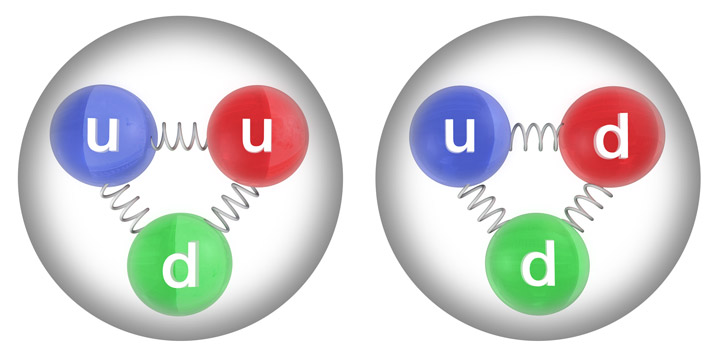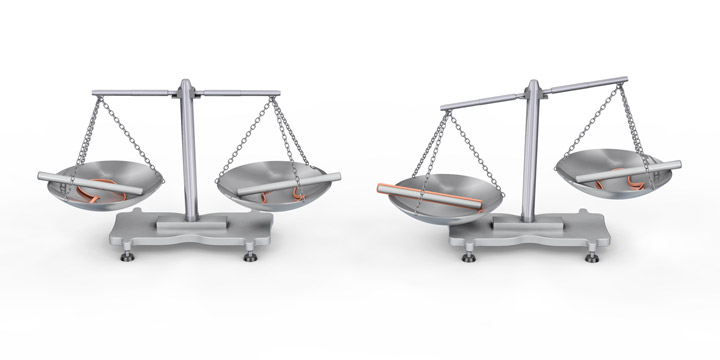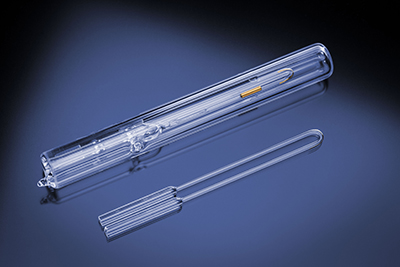4 Rates

On the Fundamentals of Mass and Density

An understanding of the properties “mass” and “density” is important for many areas of science and industry. This article briefly describes the fundamentals of mass and density as well as methods to determine them and their metrological background.

What is mass?

Isaac Newton provided an early definition of mass – “The quantity of matter is the measure of the same, arising from its density and bulk conjunctly  – in which he defined mass from density and “bulk”, the latter meaning volume. To find out what mass is requires knowledge of how atoms, out of which matter is composed, acquire mass. Atoms have a tiny nucleus and an electron shell some 100,000 times larger in diameter than the nucleus itself. The nucleus carries over 99 % of the atomic mass and comprises protons and neutrons. These consist of quarks and gluons, which are considered elementary particles (Figure 1). The gluons are the quanta of the so-called “strong nuclear force” which keeps the protons, neutrons, and the nucleus bound.Figure 1: The proton (left) and neutron (right) contain up quarks (u) and down quarks (d) and are held together by gluons (the zigzag lines). Source: commons.wikimedia.org/wiki/Quark

The mass of elementary particles

The mass of elementary particles can be attributed to their degree of interaction with the Higgs field, which is postulated to fill all of space. The quantum of the Higgs field is the Higgs boson as detected at CERN in Geneva in 2012. Particles which do not interact with the Higgs field acquire no mass and move at the speed of light, like the photon for example.

However, only a small percentage of the mass of the atomic nucleus can be attributed to the Higgs mechanism. The majority of its mass comes from the binding energy of the strong nuclear force. How binding energy contributes to the mass of the nucleus can be explained by the most prominent law of physics: Albert Einstein’s E=m*c². It says that energy E and mass m are essentially the same thing.

The mass of the atomic nucleusFigure 2: Left: A stick and a rubber ring of equal mass are placed in each pan of a beam balance; the beam is exactly horizontal. Right: By stretching one rubber ring over the stick energy gets stored in this rubber ring. Therefore, its mass and weight increase and the balance shows a weight difference. While this effect is much too small to be observable with real balances the principle holds.

To see how this relates to the question of where the majority of the mass of the atomic nucleus comes from, see Figure 2. In this illustrative example, a stick and a rubber ring are placed into each pan of a beam balance. The sticks and the rubber rings are selected to have exactly the same mass and therefore the same weight. This causes the beam of the balance to be exactly horizontal. If one of the rubber rings is stretched over the stick and this is placed into one pan while in the other pan the stick and the rubber ring remain separate the balance is (at least in principle) no longer exactly horizontal.

The stretched rubber ring has additional energy E stored and according to Einstein this adds to the mass by an amount Δm=E/c². Therefore, the mass of the stretched rubber ring is increased and the balance shows a difference in weight, at least in principle. Because the energy E is divided by c², which is the speed of light squared, the change in mass is much too small to be observable with real balances.

How the different effects contribute to the mass of the atomic nucleus

The mass of the atomic nucleus therefore originates from the following effects:

1. The strong nuclear force within the nucleus: Due to the net positive charge of its constituents a huge electrostatic force strives to blow up the nucleus. The strong nuclear force within the nucleus acts against the electrostatic force and keeps the nucleus bound. Therefore, the strong nuclear force comprises enormous amounts of binding energy, making a large contribution to the mass of the nucleus.
2. The kinetic energy arising from Heisenberg’s uncertainty principle: Additionally, because of Heisenberg’s uncertainty principle, the elementary particles in the nucleus cannot sit still, they have to buzz around at speeds equivalent to a major fraction of the speed of light. This kinetic energy results in a corresponding increase in mass and would cause the nucleus to explode were it not counteracted by the strong nuclear force. This increases the binding energy of the strong nuclear force and its mass contribution.
3. The Higgs mechanism: As described above. In fact, this mechanism contributes to the mass of the nucleus least.

How is mass determined?

Mass is determined using a balance. Balances utilize the gravitational force exerted by the planet earth on the specimen. Balances measure a force, and this force is e.g. different on the moon, while mass is entirely independent of where it is measured. The force as measured by the balance is called weight. As long as such measurements are not carried out in a vacuum chamber, they determine the weight in air of the specimen. Weight in air usually gives slightly lower values than weight in vacuum, because the specimen “floats” in air, meaning there is some buoyancy effect involved. This can be corrected for if it is required.

Converting weight into mass

Using the balance in Figure 2, the weight of a specimen can be converted into mass as follows:

1. The weight of the specimen in one pan is balanced by calibration weights of known mass in the other pan, so that the beam becomes horizontal.
2. Now the mass of the specimen agrees with the mass of the calibration weights, apart from the small air buoyancy effects which are normally ignored.
3. In this way the mass of the specimen is determined.

Modern electronic balances function in a more elaborate way but the fundamentals are the same. For traceable mass determinations, suitable mass units and calibration weights traceable to an international mass standard are required. According to the SI International System of Units the unit of mass is the kilogram [kg]. The current international mass standard is the original kilogram prototype located in Paris, France. It is a cylinder made of platinum-iridium alloy (Figure 3).

However, there were issues with the long-term stability of the mass value of the original kilogram prototype. Therefore, for decades researchers have been working to find a better mass standard which relies on basic physical properties only. These efforts seem to have been successful and it is expected that the original kilogram prototype will be replaced by a new mass standard at the 26th General Conference on Weights and Measures in November 2018.

What is density?

Density, i.e. mass density, is the mass of a specimen divided by its volume. The SI unit for density is kilograms per cubic meter [kg/m³]. As mentioned above, Newton used density as one of the defining properties of mass. While many believe that Newton’s is a circular definition, it is worth mentioning that one of the new standards proposed for replacing the original kilogram prototype is essentially based on the density of spheres made of a silicon-28 monocrystal.

How is density measured?

The density of a specimen is determined by combining the measurements of mass and volume. For the measurement of mass, see above. Volume measurement can be broken down into length measurements, for which the meter units apply. The meter is defined as the distance in vacuum a ray of light crosses in slightly more than one third of a nanosecond, so the speed of light and time are the defining properties of a meter.

Density measurement of solids

Accurately determining the volume of a solid material is a tedious procedure. Typically a pycnometer of known volume is applied with the specimen placed inside. The volume of the specimen is determined from the volume of liquid or gas it replaces in the pycnometer. Mass and volume determinations are normally carried out in separate steps and the density is computed from the two results.

Density measurement of liquidsFigure 4: A bare U-tube in front and the complete oscillating U-tube density sensor in the back. The U-tube is filled with the sample and electronically put into oscillation at its natural frequencies. The density of the sample is derived from these values.

The density of liquids is an important physical parameter which can be used to calculate concentration and in many other applications. To determine the density of liquids the following methods are used:

• Hydrostatic balance or hydrometer: a body of known volume is immersed into the sample and its weight loss due to buoyancy is determined. Buoyancy is proportional to density and can easily be converted into the latter. To achieve highly accurate measurements it is essential to accurately control and compensate for temperature, surface tension effects, etc.
• Pycnometer: a bottle (pycnometer) of known volume is filled with the sample liquid. The weight difference between the filled and the empty pycnometer is determined. From that and the known pycnometer volume the density is calculated.
• Oscillating U-tube density meter: Due to their speed and accuracy these are the most frequently applied instruments for density measurements. The amount of sample required is much lower than for the above two methods. The U-tube’s inner volume defines the measured sample volume. It is filled with the sample and set into oscillation at its natural frequencies by electronic means. The natural frequencies depend upon the mass of the filled in sample and are automatically converted into the sample’s density. Figure 4 shows an example of an oscillating U-tube density sensor.

For a detailed explanation of the technology used by oscillating density meters, see here.

Conclusion

The article describes the fundamentals of mass and density. It starts with an explanation of how the property mass comes about at the level of the atomic nucleus. Then methods to determine mass and density and their metrological background are described. Finally an outlook is given on how the metrological basis of these measurements will change in the near future.